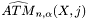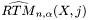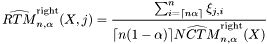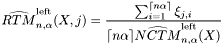#Boost C++ Libraries

...one of the most highly regarded and expertly designed C++ library projects in the world.

## Struct template tail_variate_means_impl

boost::accumulators::impl::tail_variate_means_impl — Estimation of the absolute and relative tail variate means (for both left and right tails).

## Synopsis

```// In header: <boost/accumulators/statistics/tail_variate_means.hpp>

template<typename Sample, typename Impl, typename LeftRight,
typename VariateTag>
struct tail_variate_means_impl {
// types
typedef numeric::functional::average< Sample, std::size_t >::result_type float_type;
typedef std::vector< float_type >                                        array_type;
typedef iterator_range< typename array_type::iterator >                  result_type;

// construct/copy/destruct
tail_variate_means_impl(dont_care);

// public member functions
template<typename Args> result_type result(Args const &) const;
};```

## Description

For all-th variates associated to thelargest samples (or thesmallest samples in case of the left tail), the absolute tail meansare computed and returned as an iterator range. Alternatively, the relative tail meansare returned, which are the absolute tail means normalized with the (non-coherent) sample tail mean.

Equation 1.17.Equation 1.18.Equation 1.19.Equation 1.20.### `tail_variate_means_impl` public construct/copy/destruct

1. `tail_variate_means_impl(dont_care);`

### `tail_variate_means_impl` public member functions

1. `template<typename Args> result_type result(Args const & args) const;`